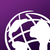# Perform Raster Calculation from Multiple Sub-folder Using ArcPy

1635
23
10-07-2016 05:14 AMOccasional Contributor III

I have five subfolders with Rasters name with the same parameter. I am trying to figure out a way to Loop average of all  raster and save output raster in outfolder . For instance

``````Subfolder1   Subfolder2   Subfolder3   Subfolder4    Subfolder5   Outfolder
r001_NPP.tif r001_npp.tif r001_NPP.tif r_001_NPP.tif r001_NPP.tif r001_MEAN_NPP.tif‍‍‍‍‍‍

‍‍‍‍‍‍‍``````

I have tried to write code for this, Below i post my code what i have so far

``````import arcpy, os, glob
arcpy.CheckOutExtension("Spatial")

ws1 = glob.glob(r'D:\subfolder1\*.tif')
ws2 = glob.glob(r'D:\subfolder2\*.tif')
ws3 = glob.glob(r'D:\subfolder3\*.tif')
ws4 = glob.glob(r'D:\subfolder4\*.tif')
ws5 = glob.glob(r'D:\subfolder5\*.tif')
outws = r'D:\outfolder'

for r in ws1, ws2, ws3, ws4:
basename = os.path.basename(r).split("_")
r1 = arcpy.sa.Raster(os.path.join(ws1, basename + "r_NPP.tif"))
r2 = arcpy.sa.Raster(os.path.join(ws2, basename + "r_NPP.tif"))
r3 = arcpy.sa.Raster(os.path.join(ws3, basename + "r_NPP.tif"))
r4 = arcpy.sa.Raster(os.path.join(ws4, basename + "r_NPP.tif"))
r5 = arcpy.sa.Raster(os.path.join(ws5, basename + "r_NPP.tif"))

result = (r1 + r2 + r3 + r4 + r5) / 5
outname = basename + "r_Mean_NPP.tif"

result.save(os.path.join(outws, outname))‍‍‍‍‍‍‍‍‍‍‍‍‍‍‍‍‍‍‍‍‍‍‍‍‍‍‍‍‍‍‍‍‍‍‍‍‍‍‍‍‍‍‍‍‍‍‍‍‍‍‍‍‍‍‍‍‍‍‍‍‍‍‍‍‍‍‍‍‍‍‍‍‍‍‍‍‍‍‍‍‍‍‍‍‍‍‍‍‍‍‍‍‍‍‍‍‍‍‍‍‍‍‍‍‍‍‍‍‍‍‍‍‍‍‍‍‍‍‍‍‍‍‍‍‍‍‍‍‍‍‍‍‍‍‍‍``````
Tags (2)
1 Solution

Accepted SolutionsbyEsri Esteemed Contributor

I can't reproduce the error you mentioned. I did encounter some other errors that should be corrected like:

• include import os at beginning of script and
• change "out_workplace" into "out_workspace"

``````import arcpy
import os
arcpy.CheckOutExtension("spatial")

# generate list of rasters
##workspace = r"D:\TEST"
##out_workplace = r"D:\TEST\MEAN_NPP"
workspace = r"C:\GeoNet\MeanRasters\Case20161009"
out_workspace = r"C:\GeoNet\MeanRasters\Case20161009\MEAN_NPP"

for ras_num in [str(i).zfill(3) for i in range(1, 13)]:
print ras_num
rasters = []
walk = arcpy.da.Walk(workspace, datatype="RasterDataset", type="TIF")
for dirpath, dirnames, filenames in walk:
for filename in filenames:
if ras_num in filename:
rasters.append(os.path.join(dirpath, filename))

# calculate mean
if len(rasters) > 0:
print "Calculating mean of rasters: ", ', '.join(rasters)
ras_mean = arcpy.sa.CellStatistics(rasters, "MEAN")
ras_mean.save(os.path.join(out_workspace, 'r{0}_MEAN_NPP.TIF'.format(ras_num)))
else:
print "no rasters for ras_num", ras_num‍‍‍‍‍‍‍‍‍‍‍‍‍‍‍‍‍‍‍‍‍‍‍‍‍‍‍‍‍‍‍‍‍‍‍‍‍‍‍‍‍‍‍‍‍‍‍‍‍‍‍‍``````

This created the mean raster for ras_num 001 to 005, see attached ZIP with resulting rasters

23 RepliesbyMVP Esteemed Contributor

I would look into arcpy.da.walk() to generate the list of rasters, and use the Spatial Analyst Cell Statistics tool on the raster list.

Cell Statistics is much more efficient, especially with long lists of rasters -- and it also has a very handy DATA/NODATA option so null is treated as zero in the cell by cell summation.

Untested code:

``````import arcpy
arcpy.CheckOutExtension("spatial")
from arcpy.sa import *

# generate list of rasters
workspace = r"D:\"
rasters = []
walk = arcpy.da.Walk(workspace, datatype="RasterDataset", type="TIF")
for dirpath, dirnames, filenames in walk:
for filename in filenames:
rasters.append(os.path.join(dirpath, filename))

# calculate mean
sum = CellStatistics(rasters, "MEAN")
sum.save(os.path.join(out_workspace, "npp_sum.tif")‍‍‍‍‍‍‍‍‍‍‍‍‍‍‍‍‍‍‍‍‍‍‍‍‍‍``````Occasional Contributor III

Thank you. I have updated my suggested by you, problem still persist, i am not understanding how to iterate through each folder, take all series of 001_NPP from all folder and calculate average oh them and save it as 001_NPP_MEAN, below is my updated code and along with code i have attached reference raster also which i am using for my raster calculation,

``````import arcpy
arcpy.CheckOutExtension("spatial")
from arcpy.sa import *

# generate list of rasters
workspace = r"D:\TEST"
out_workplace = "D:\TEST\MEAN_NPP"

rasters = []
walk = arcpy.da.Walk(workspace, datatype="RasterDataset", type="TIF")
for dirpath, dirnames, filenames in walk:
for filename in filenames:
rasters.append(os.path.join(dirpath, filename))

# calculate mean
sum = CellStatistics(rasters, "MEAN")
sum.save(os.path.join(out_workspace, "npp_sum.tif")‍‍‍‍‍‍‍‍‍‍‍‍‍‍‍‍‍‍‍‍‍‍‍‍‍‍‍‍‍‍‍‍‍‍‍‍‍‍‍‍‍‍‍‍‍‍‍‍‍‍‍‍‍‍‍‍‍‍‍‍‍‍‍‍‍‍‍‍``````byMVP Esteemed Contributor

your code is only minimally updated from what Neil provided and it was untested.

What was the error message?

issues to get you started...

• Line 13 is not indented properly
• Walk—Help | ArcGIS for Desktop you need to check the correct format
• datatype and type in Walk are incorrect
• you shouldn't use from X import *

You need to provide some input to the solution and to do that providing commentary as to what went wrong and where would go a long wayOccasional Contributor III

Dan . thanks , you are right. I didn't post the error massage. Line no 13 i am getting the Indentation Error massage. I tried to fix it but not solved yet. error massage below

``````File "<module1>", line 13
rasters.append(os.path.join(dirpath, filename))
^
IndentationError: expected an indented block
‍‍‍‍``````byMVP Esteemed Contributor

Ok.... add four (4) more spaces to the beginning of line 13 so it is indented properly

Here is the python style guide recommendations PEP 8 -- Style Guide for Python Code | Python.orgOccasional Contributor III

Dan , thanks . I have solved the indentation error, and i updated my code but still i could not solve the problem, updated code below

error masage

``````ile "<module1>", line 18
sum.save(os.path.join(out_workspace, 'r{0}_MEAN_NPP.TIF'.format(ras_num)
^
SyntaxError: invalid syntax‍‍‍‍``````

Updated Code

``````import arcpy
arcpy.CheckOutExtension("spatial")
from arcpy.sa import *

# generate list of rasters
workspace = r"D:\TEST"
out_workplace = "D:\TEST\MEAN_NPP"

for ras_num in [str(i).zfill(3) for i in range(1, 12)]:
rasters = []
walk = arcpy.da.Walk(workspace, datatype="RasterDataset", type="TIF")
for dirpath, dirnames, filenames in walk:
for filename in filenames:
rasters.append(os.path.join(dirpath, filename))

# calculate mean
sum = CellStatistics(rasters, "MEAN")
sum.save(os.path.join(out_workspace, 'r{0}_MEAN_NPP.TIF'.format(ras_num)‍‍‍‍‍‍‍‍‍‍‍‍‍‍‍‍‍‍``````byMVP Esteemed Contributor

Change line 7 to

out_workplace = r"D:\TEST\MEAN_NPP"

You missed the "r" in that path.byEsri Esteemed Contributor

In addition, line 18 is missing 2 closing brackets "))" at the end of the line. Perhaps you didn't copy the entire line.byMVP Esteemed Contributor

Hey no fair. ;)